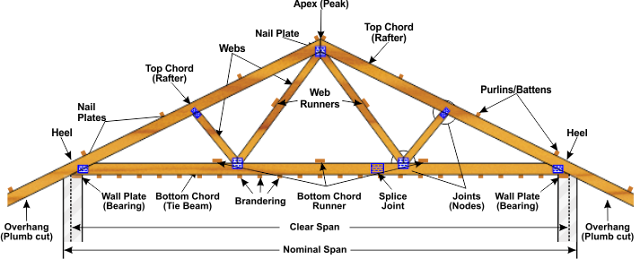# Design Of Roof Truss:If load from purlin, false ceiling etc are applied in between the nodes, then principal rafters or main ties are designed for combined stresses from bending and axial loads.

IS 800 - 2007 provides general design procedure for the angle Purlins conforming to steel grade of Fe 410 of O, S, W  and not exceeding slope more than 30 degree based on live load of 750 N/m2.

If spacing of Truss is 4 m and pitch of truss is 1:4 then self weight of the truss is taken as,
w = (l/3+6) of plan area .... in kN/m2.
Then add all the dead loads with this such as GI sheet, AC sheet and Purlins weight.
Then multiply with the plan area to get the actual DL.

If the angle is less than 10 degree, then the LL will be directly taken as 750 N/m2.
If slope of truss is more than 10 degree, live load is taken as
LL = 0.75 - 0.02( actual angle - 10) ... in kN/m2.
Then multiply with plan area to get the LL.

In wind load we need to find,
Vz = Vb.k1.k2.k3.k4
k1 is risk coefficient
k2 is terrian, height, structure size factor
k3 is topography factor
k4 is usually taken as 1
All of the above factors can be found from the IS 875 Part 3, Table 4 and Table 5.

Now, F = (Cep-Cpi).A.Pd
where,
Cpe = External Pressure Coefficient (Towards)
Cpi = Internal Pressure Coefficient (Away)
A = Surface Area of Structural Element
Pd = Pz = Design Wind Pressure in N/m2.

#### For Cpe:

Get it from Table 8 in the code

#### For Cpi:

For Low permeability (less than 5% opening in wall area) = +-0.2
For Medium permeability ( 5- 20 % ) = +-0.5
For Large permeability  (>20%) = +-0.7

The calculate the final WL.

1.I generally check this kind of article and I found your article which is related to my interest. Genuinely, it is good and instructive information about roof truss. Thanks for sharing an amazing article here. Dry Ice Blasting

2.Thank You so much for your comment. I share such short and on point blogs, do check then all.

3.Excellent information, This information will always help everyone for gaining knowledge. So please always share your valuable information. I am very thankful to you for providing good information. dry ice blasting services